The correct expression derived for the energy of an electron in the nth energy level is for H-atom

1.

2.

3.

4.

Difficulty Level:

For any H like system, the ratio of velocities of I, II & III orbit i.e.,  will be

1.  1 : 2 : 3

2.  1 : 1/2 : 1/3

3.  3 : 2 : 1

4.  1 : 1 : 1

Difficulty Level:

Select the incorrect graph for velocity of ${\mathrm{e}}^{-}$ in an orbit :

1.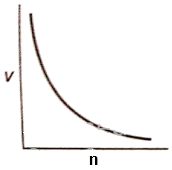2.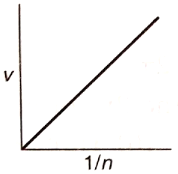3.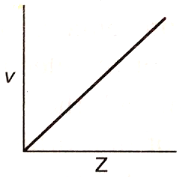4.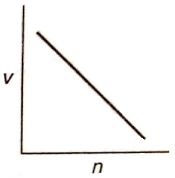Difficulty Level:

Number of waves produced by an electron in one complete revolution in nth orbit is:

1.  $\mathrm{n}$

2.  ${\mathrm{n}}^{2}$

3.  $\left(\mathrm{n}+1\right)$

4.  $\left(2\mathrm{n}+1\right)$

High Yielding Test Series + Question Bank - NEET 2020

Difficulty Level:

The energy of an electron moving in nth Bohr's orbit of an element is given by ${\mathrm{E}}_{\mathrm{n}}=\frac{-13.6}{{\mathrm{n}}^{2}}{\mathrm{Z}}^{2}$ eV/atom (Z=atomic number). The graph of  (keeping "n" constant) will be:

1.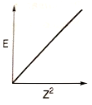2.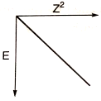3.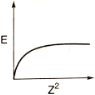4.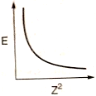High Yielding Test Series + Question Bank - NEET 2020

Difficulty Level:

The spectrum produced from an element is:

1.  atomic spectrum

2.  line spectrum

3.  absorption spectrum

4.  any one of the above

Difficulty Level:

Which of the following expressions represents the spectrum of Balmer series (If n is the principal quantum number of higher energy level) in Hydrogen atom?

1.

2.

3.

4.

Difficulty Level:

The H-spectrum show:

1.  Heisenberg's uncertainty principle

2.  Diffraction

3.  Polarization

4.  Presence of quantized energy level

Difficulty Level:

In photoelectric effect, the kinetic energy of photoelectrons increases linearly with the

1.  Wavelength of incident light

2.  Frequency of incident light

3.  Velocity of incident light

4.  Atomic mass of an element

Difficulty Level:

Slope of ${\mathrm{V}}_{0}$ vs v curve is (where ${\mathrm{V}}_{0}$=stopping potential, v=subjected frequency)

1.  $\mathrm{e}$

2.  $\frac{\mathrm{h}}{\mathrm{e}}$

3.  $\mathrm{\varphi }$

4.  $\mathrm{h}$